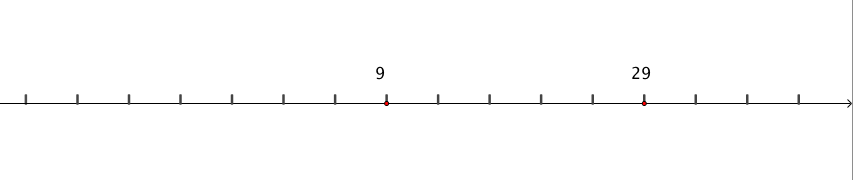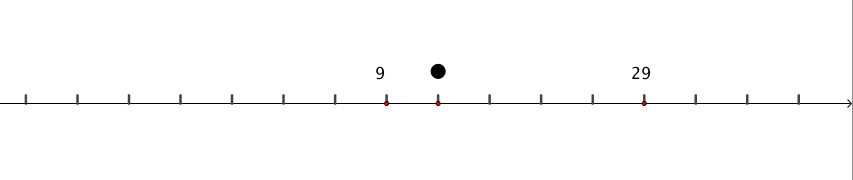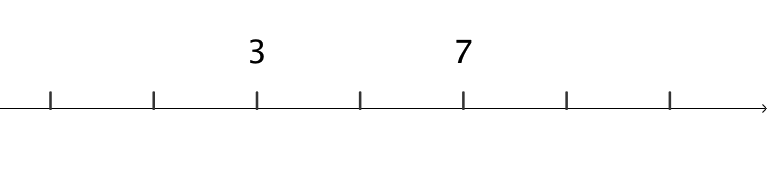#### You may also like### Worms

Place this "worm" on the 100 square and find the total of the four squares it covers. Keeping its head in the same place, what other totals can you make?### Greetings

From a group of any 4 students in a class of 30, each has exchanged Christmas cards with the other three. Show that some students have exchanged cards with all the other students in the class. How many such students are there?### Writ Large

Suppose you had to begin the never ending task of writing out the natural numbers: 1, 2, 3, 4, 5.... and so on. What would be the 1000th digit you would write down.

# Number Lines in Disguise

##### Age 7 to 14 Challenge Level:Click below to reveal some questions you might like to think about.

What number would be where the dot is?
How do you know?Where would $0$ be on the line?

Often if a mathematician is stuck they might try an example with smaller numbers. Click below to reveal another example.

Consider the number line below.Can you figure out what any of the other numbers are on the number line?

Where would $0$ be on this line?

What strategies did you use?

Below is an interactive number line. Can you work out what number is marked by the dot? Once you think you know, reveal the answer to check if you were right.

Explore the different number lines at different levels. Think about the strategies that you might use if you get stuck on the harder levels.

You could use this worksheet to create your own challenges.

What makes a challenge easier or harder?

We would love to hear about the strategies you used to figure out the missing numbers, and how you persevered and showed resilience if you got stuck!